# Reachability Schedulability and Optimality Ansgar Fehnker June 3

• Slides: 45
Download presentation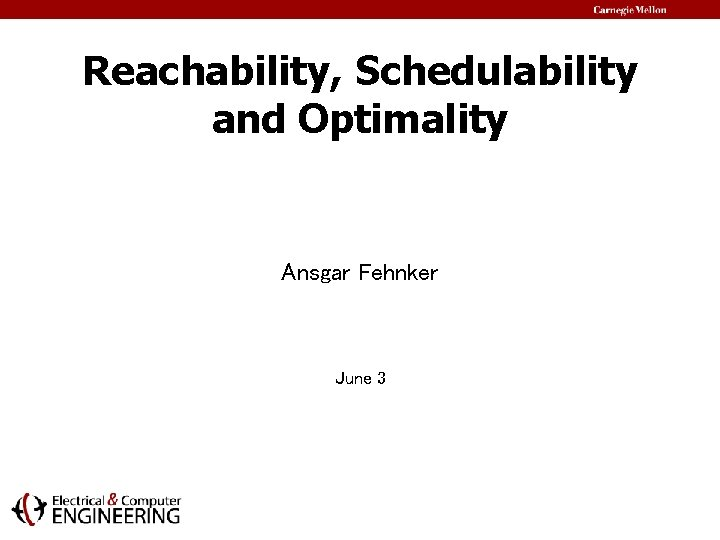Reachability, Schedulability and Optimality Ansgar Fehnker June 3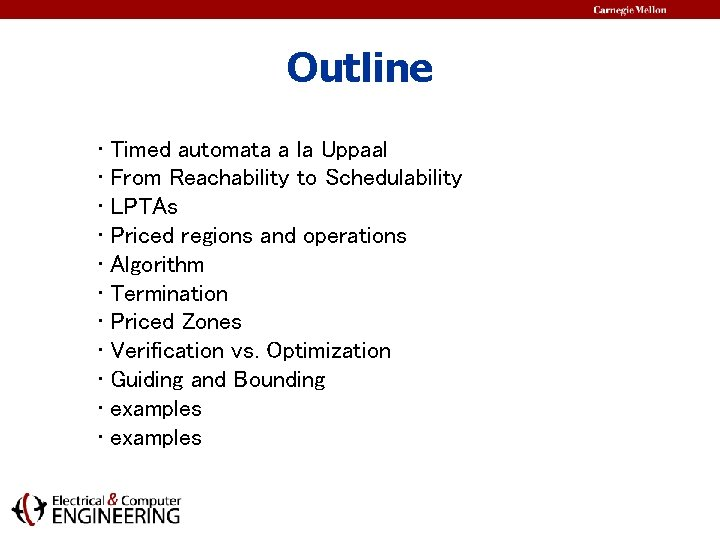Outline • Timed automata a la Uppaal • From Reachability to Schedulability • LPTAs • Priced regions and operations • Algorithm • Termination • Priced Zones • Verification vs. Optimization • Guiding and Bounding • examplesTimed Automata (UPPAAL) • Network of Automata – Synchronization (CCS-like) a! a?Timed Automata (UPPAAL) • Network of Automata x 7 – Synchronization (CCS-like) • Clocks in description 3 x 7 a! y>4 a? y: =0 – Time passes uniformly – Guard/reset on action - Invariants on location Uppaal is a modelchecker for. Timed Automata with emphasis on reachability properties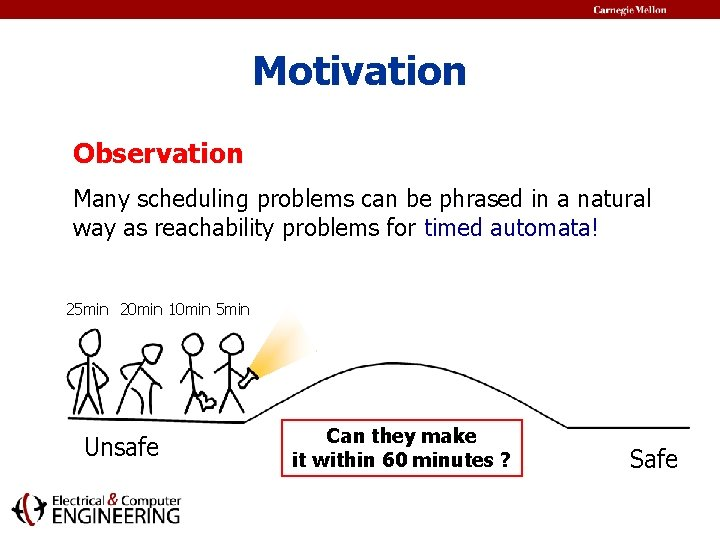Motivation Observation Many scheduling problems can be phrased in a natural way as reachability problems for timed automata! 25 min 20 min 10 min 5 min Unsafe Can they make it within 60 minutes ? Safe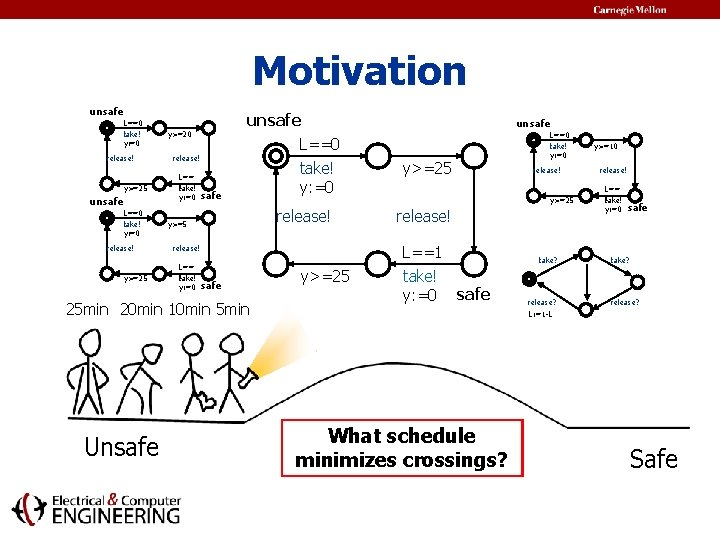Motivation unsafe L==0 take! y: =0 release! y>=25 unsafe y>=20 release! L== t 1 ake! y: =0 safe L==0 take! y: =0 release! y>=5 L== t 1 ake! y: =0 safe L==0 take! y: =0 y>=25 release! L==1 release! 25 min 20 min 10 min 5 min Unsafe unsafe y>=25 take! y: =0 take? safe What Canschedule is they schedule themake fastest miniit minimizes within schedule? unsafe 60 crossings? minutes time? ? release? L: =1 -L y>=10 release! L== 1 take! y: =0 safe take? release? Safe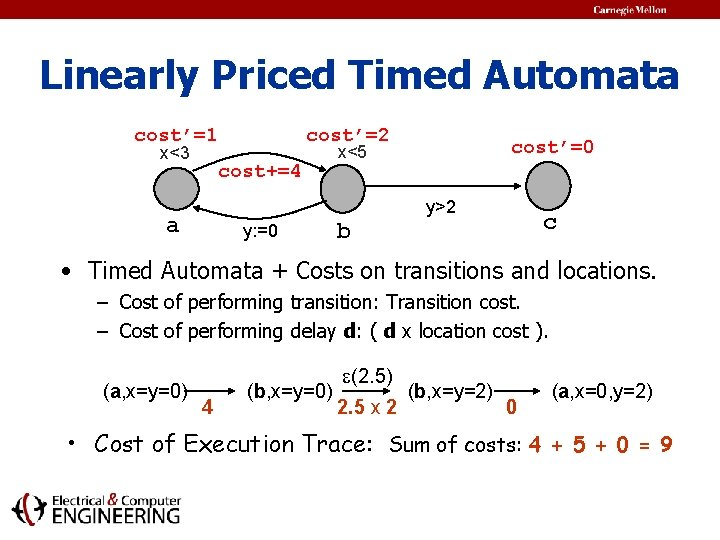Linearly Priced Timed Automata cost’=1 x<3 cost’=2 cost+=4 cost’=0 x<5 y>2 a y: =0 c b • Timed Automata + Costs on transitions and locations. – Cost of performing transition: Transition cost. – Cost of performing delay d: ( d x location cost ). (a, x=y=0) 4 (b, x=y=0) (2. 5) 2. 5 x 2 (b, x=y=2) 0 (a, x=0, y=2) • Cost of Execution Trace: Sum of costs: 4 + 5 + 0 = 9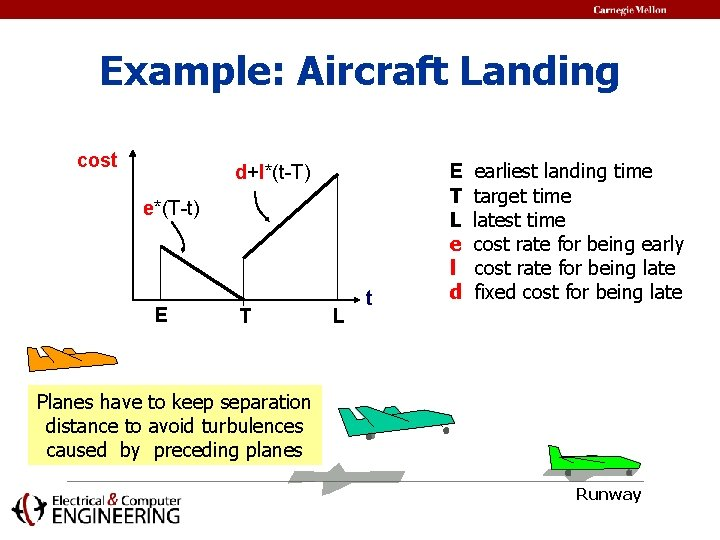Example: Aircraft Landing cost d+l*(t-T) e*(T-t) E T L t E T L e l d earliest landing time target time latest time cost rate for being early cost rate for being late fixed cost for being late Planes have to keep separation distance to avoid turbulences caused by preceding planes RunwayExample: Aircraft Landing x <= 5 x >= 4 land! x <= 5 cost’=3 x=5 cost+=2 x <= 9 cost’=1 land! 4 5 9 3 1 2 earliest landing time target time latest time cost rate for being early cost rate for being late fixed cost for being late Planes have to keep separation distance to avoid turbulences caused by preceding planes Runway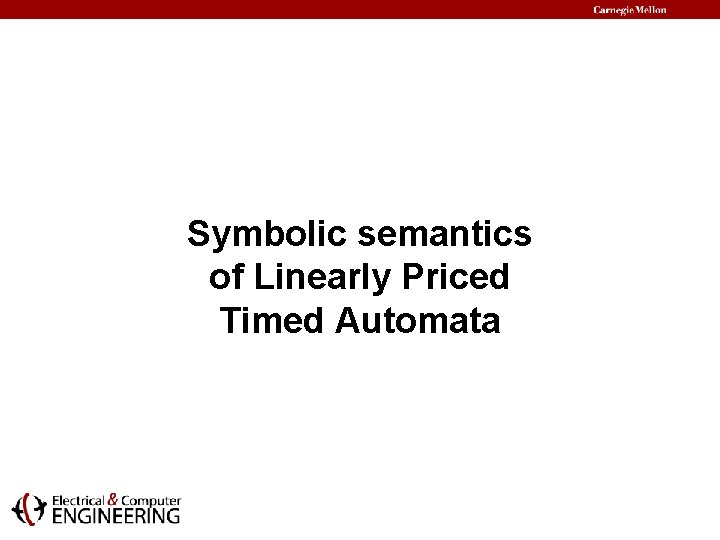Symbolic semantics of Linearly Priced Timed AutomataZones Basic idea: Define a delay and reset over zones 1 y 4 0 x 3 -2 x-y 0 y delay 1 y 0 x -2 x-y 0 x x<3 y>2 a y: =0 b c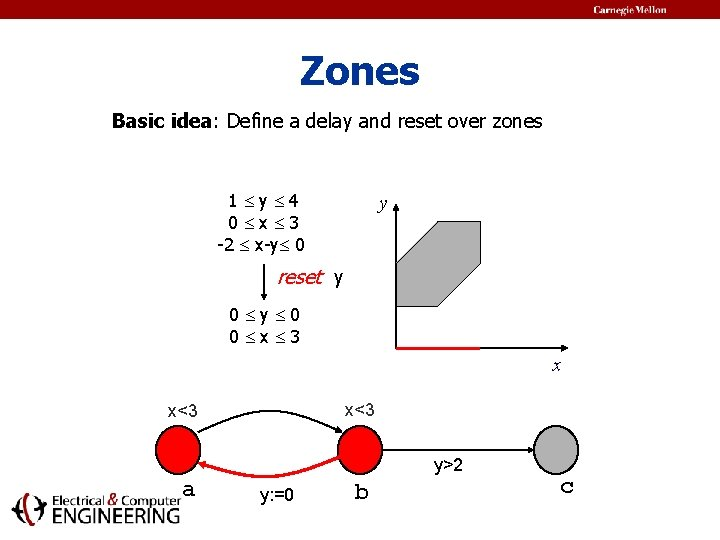Zones Basic idea: Define a delay and reset over zones 1 y 4 0 x 3 -2 x-y 0 y reset y 0 0 x 3 x x<3 y>2 a y: =0 b c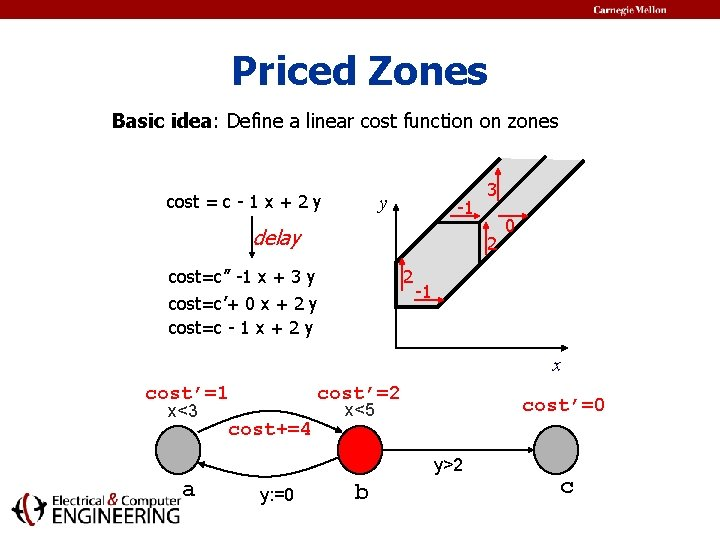Priced Zones Basic idea: Define a linear cost function on zones cost = c - 1 x + 2 y y -1 delay 3 2 2 cost=c’’ -1 x + 3 y cost=c’+ 0 x + 2 y cost=c - 1 x + 2 y 0 -1 x cost’=1 x<3 cost’=2 cost+=4 cost’=0 x<5 y>2 a y: =0 b c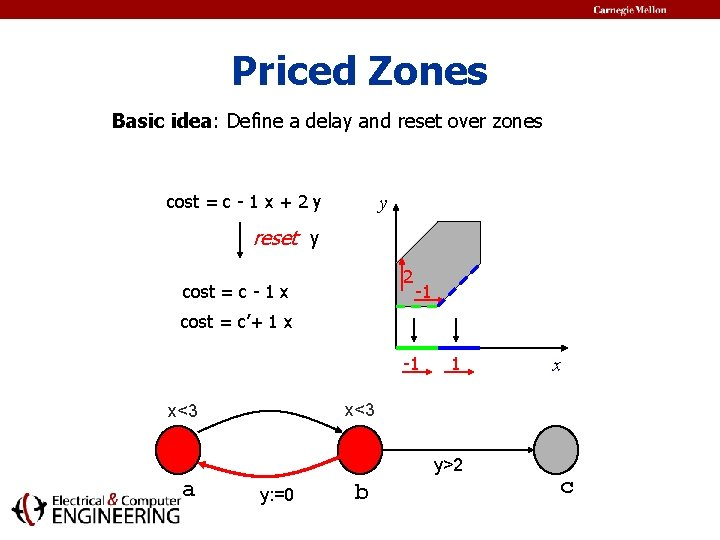Priced Zones Basic idea: Define a delay and reset over zones cost = c - 1 x + 2 y y reset y 2 cost = c - 1 x -1 cost = c’+ 1 x -1 1 x<3 y>2 a x y: =0 b c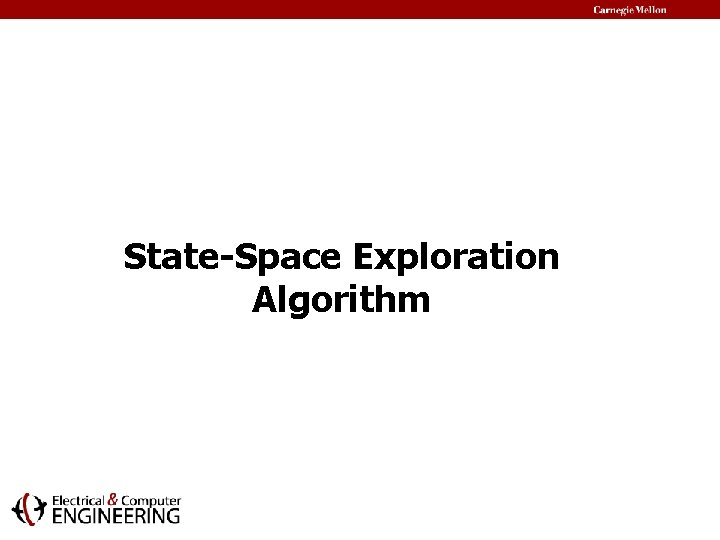State-Space Exploration AlgorithmAn Algorithm • State-Space Exploration + Use of global variable Cost. • Updated Cost whenever goal state with min( C ) <Cost is found: Cost=80 80 60 Cost=60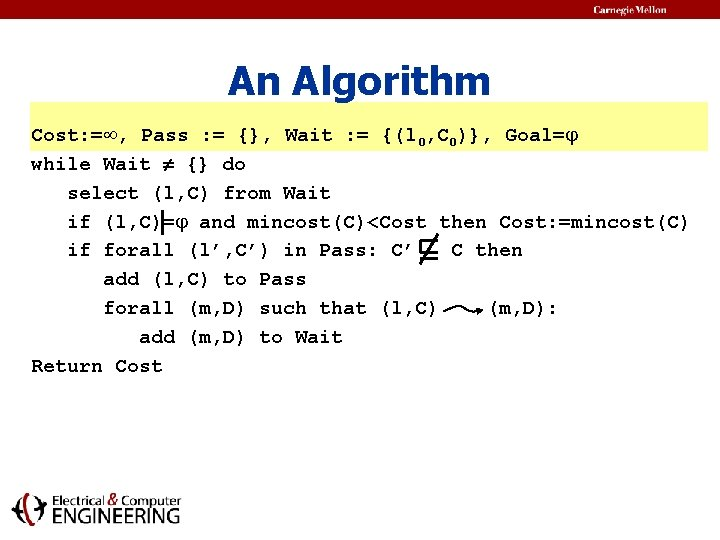An Algorithm Cost: = , Pass : = {}, Wait : = {(l 0, C 0)}, Goal= while Wait {} do select (l, C) from Wait if (l, C)= and mincost(C)<Cost then Cost: =mincost(C) if forall (l’, C’) in Pass: C’ C then add (l, C) to Pass forall (m, D) such that (l, C) (m, D): add (m, D) to Wait Return CostAn Algorithm Cost: = , Pass : = {}, Wait : = {(l 0, C 0)}, Goal= while Wait {} do select (l, C) from Wait if (l, C)= and mincost(C)<Cost then Cost: =mincost(C) if forall (l’, C’) in Pass: C’ C then add (l, C) to Pass forall (m, D) such that (l, C) (m, D): add (m, D) to Wait Return Cost Performs: symbolic operations Delay, Conjunction, and Reset of clocks.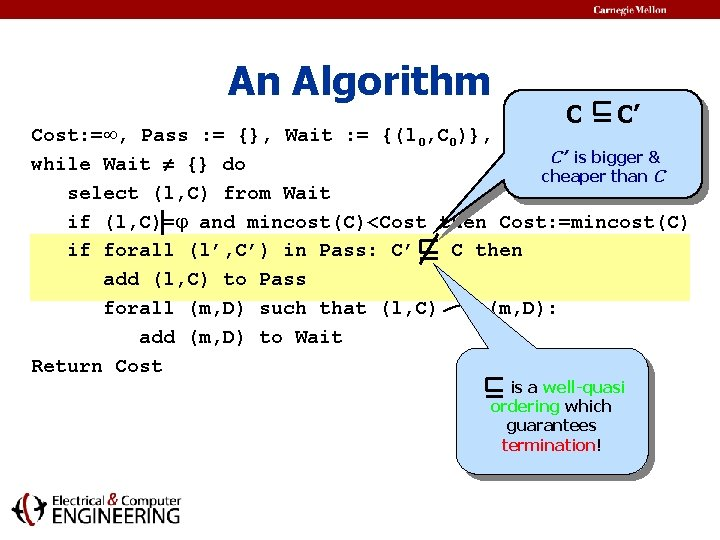An Algorithm C C’ Cost: = , Pass : = {}, Wait : = {(l 0, C 0)}, Goal= C’ is bigger & while Wait {} do cheaper than C select (l, C) from Wait if (l, C)= and mincost(C)<Cost then Cost: =mincost(C) if forall (l’, C’) in Pass: C’ C then add (l, C) to Pass. forall (m, D) such that (l, C) (m, D): add (m, D) to Wait Return Cost is a well-quasi ordering which guarantees termination!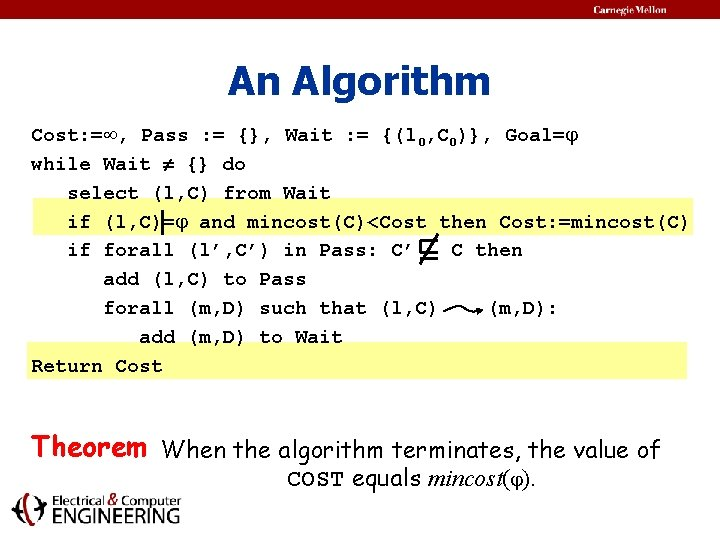An Algorithm Cost: = , Pass : = {}, Wait : = {(l 0, C 0)}, Goal= while Wait {} do select (l, C) from Wait if (l, C)= and mincost(C)<Cost then Cost: =mincost(C) if forall (l’, C’) in Pass: C’ C then add (l, C) to Pass forall (m, D) such that (l, C) (m, D): add (m, D) to Wait Return Cost Theorem When the algorithm terminates, the value of COST equals mincost( ).Efficient Reachability of LPTAs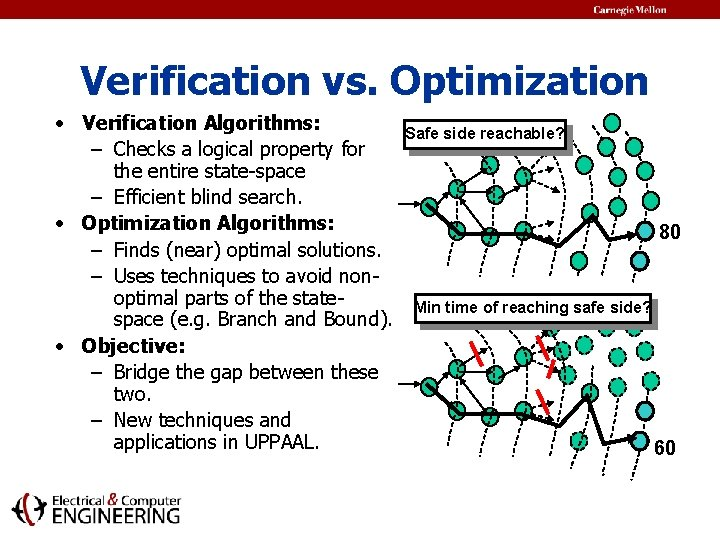Verification vs. Optimization • Verification Algorithms: – Checks a logical property for the entire state-space – Efficient blind search. • Optimization Algorithms: – Finds (near) optimal solutions. – Uses techniques to avoid nonoptimal parts of the statespace (e. g. Branch and Bound). • Objective: – Bridge the gap between these two. – New techniques and applications in UPPAAL. Safe side reachable? 80 Min time of reaching safe side? 60Minimum-Cost Order • The basic algorithm finds the minimum cost trace. • Breadth or Depth-first search -order. • Problem: Searches the entire state-space. • Minimum-Cost Search Order: Always explore state with smallest minimum cost first.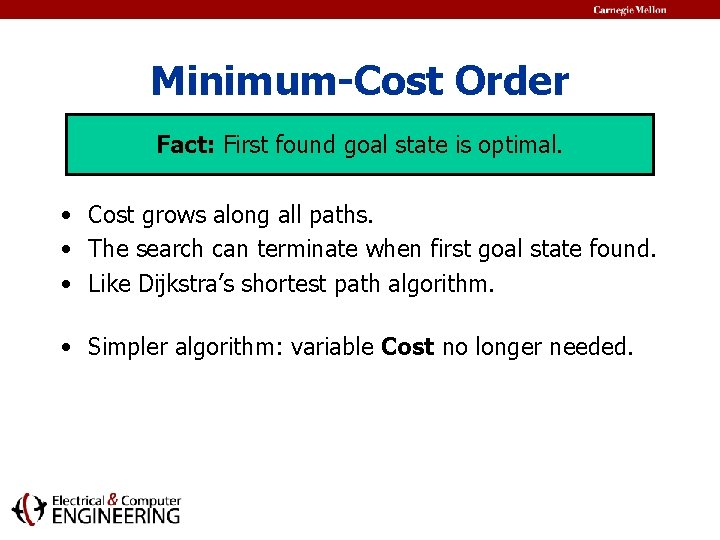Minimum-Cost Order Fact: First found goal state is optimal. • Cost grows along all paths. • The search can terminate when first goal state found. • Like Dijkstra’s shortest path algorithm. • Simpler algorithm: variable Cost no longer needed.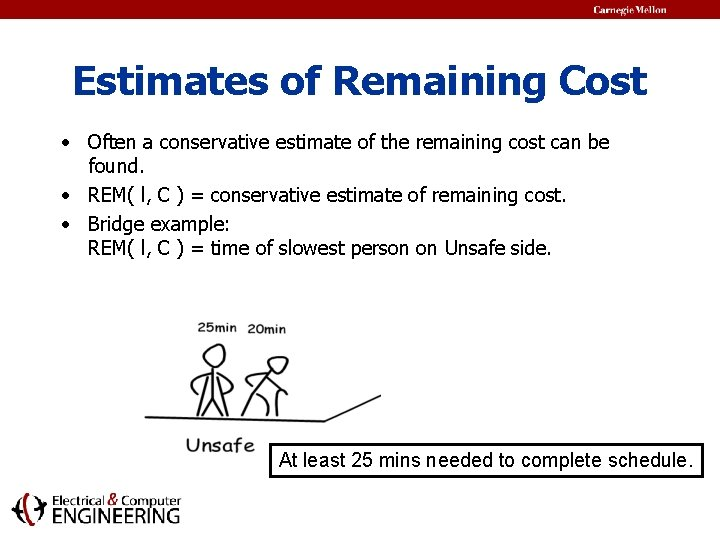Estimates of Remaining Cost • Often a conservative estimate of the remaining cost can be found. • REM( l, C ) = conservative estimate of remaining cost. • Bridge example: REM( l, C ) = time of slowest person on Unsafe side. At least 25 mins needed to complete schedule.Estimates of Remaining Cost • Basic Algorithm + Estimate of remaining cost: Only states with (min(C) + REM(l, C)) < Cost are further explored. Cost=80 min( C )+ REM ( l, C ) 80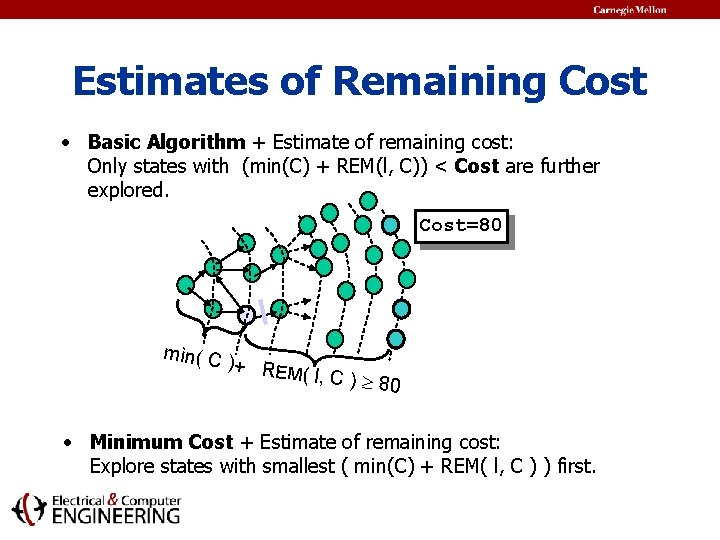Estimates of Remaining Cost • Basic Algorithm + Estimate of remaining cost: Only states with (min(C) + REM(l, C)) < Cost are further explored. Cost=80 min( C )+ REM ( l, C ) 80 • Minimum Cost + Estimate of remaining cost: Explore states with smallest ( min(C) + REM( l, C ) ) first.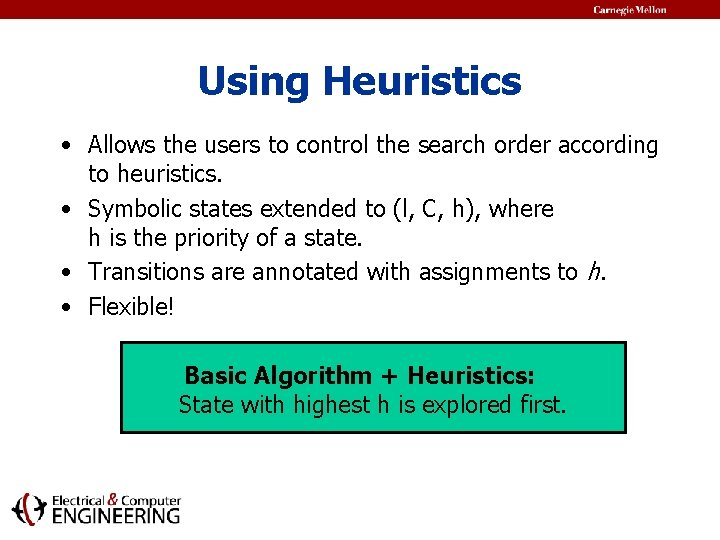Using Heuristics • Allows the users to control the search order according to heuristics. • Symbolic states extended to (l, C, h), where h is the priority of a state. • Transitions are annotated with assignments to h. • Flexible! Basic Algorithm + Heuristics: State with highest h is explored first.ExamplesUsing Heuristics Try to schedule planes in the order of their preferred landing times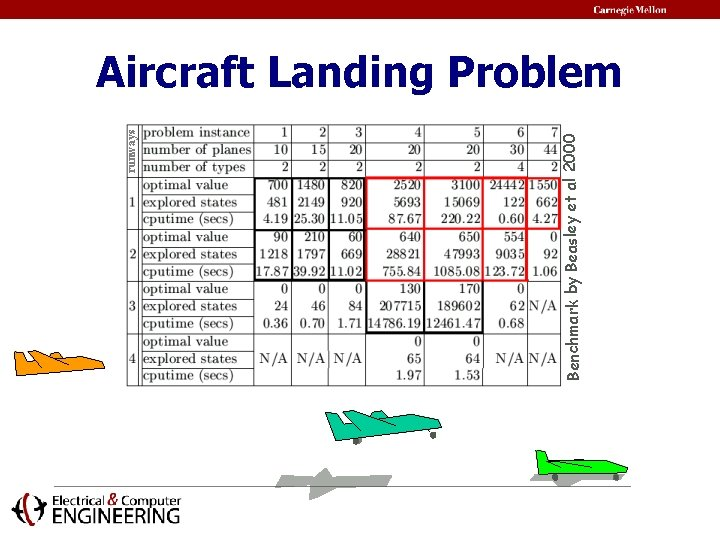Benchmark by Beasley et al 2000 runways Aircraft Landing Problem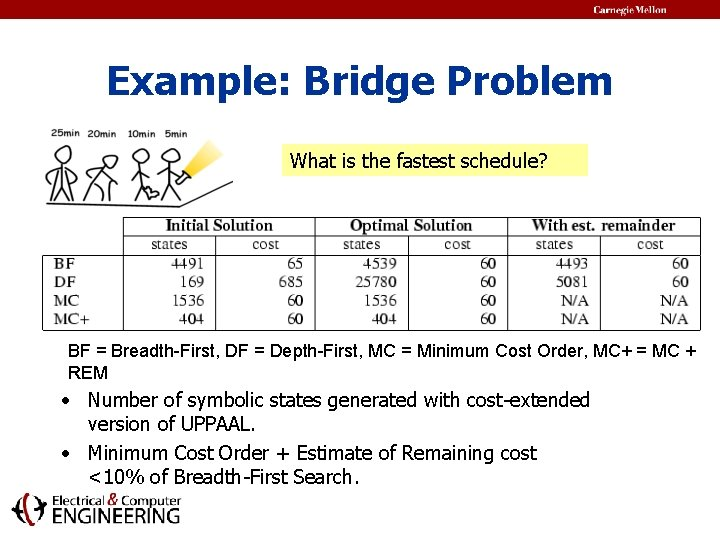Example: Bridge Problem What is the fastest schedule? BF = Breadth-First, DF = Depth-First, MC = Minimum Cost Order, MC+ = MC + REM • Number of symbolic states generated with cost-extended version of UPPAAL. • Minimum Cost Order + Estimate of Remaining cost <10% of Breadth-First Search.SIDMAR Steel Production Plant Crane A Machine 1 • A. Fehnker [RTCSA 99], T. Hune, K. G. Larsen, P. Pettersson [DSV 00] Machine 4 • Case study of Esprit-LTR project 26270 VHS • Physical plant of SIDMAR located in Gent, Belgium. Crane B • Part between blast furnace and hot rolling mill. Machine 2 Machine 3 Machine 5 Lane 2 Buffer Storage Place Objective: model the plant, obtain schedule and control program for plant. Continuos Casting Machine Lane 1SIDMAR Steel Production Plant Crane A Input: sequence of steel loads (“pigs”). @10 2 Machine 4 Load follows Recipe to obtain certain quality, e. g: start; T [email protected]; T [email protected]; T [email protected]; T [email protected]; end within 120. Machine 2 Machine 1 15 2 @20 Machine 3 2 Machine 5 @10 Lane 2 16 Buffer Crane B =127 Good schedules for ten batches within seconds, rather than bad schedules for five Output: sequence of batches within almost an higher hour. quality steel. Storage Place @40 Continuos Casting Machine @10 Lane 1SIDMAR Steel Production Plant • LEGO RCX Mindstorms. • Local controllers with control programs. • IR protocol for remote invocation of programs. • Central controller. m 1 crane a m 4 m 2 m 3 m 5 crane b buffer storage casting central controller Synthesis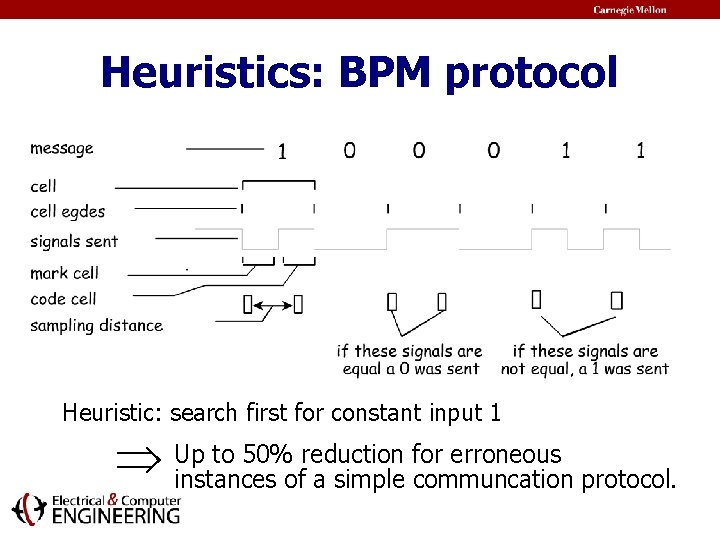Heuristics: BPM protocol Heuristic: search first for constant input 1 to 50% reduction for erroneous Up instances of a simple communcation protocol.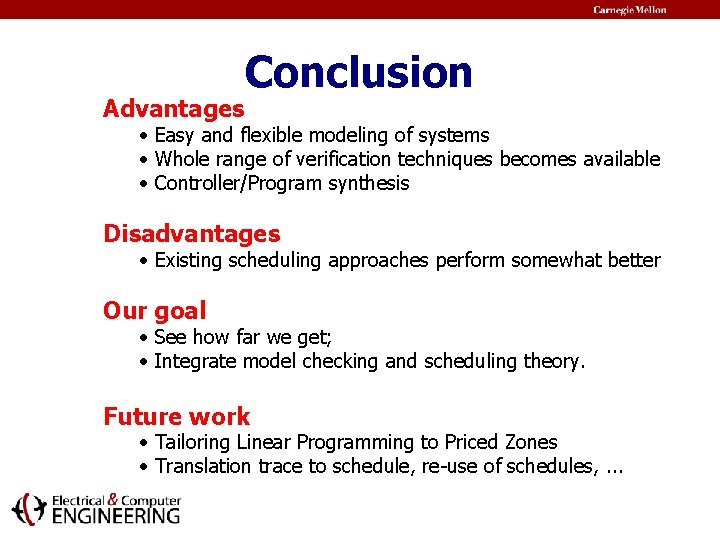Advantages Conclusion • Easy and flexible modeling of systems • Whole range of verification techniques becomes available • Controller/Program synthesis Disadvantages • Existing scheduling approaches perform somewhat better Our goal • See how far we get; • Integrate model checking and scheduling theory. Future work • Tailoring Linear Programming to Priced Zones • Translation trace to schedule, re-use of schedules, . . .Related Work • Alur, Courcourbetis, Henzinger (1993) Accumulated delays in Realtime Systems • Alur, Torre, Pappas (HSCC’ 01) Optimal Paths in Weighted Timed Automata • Behrmann, Fehnker, et all (HSCC’ 01) Minimum-Cost Reachability for Priced Timed Automata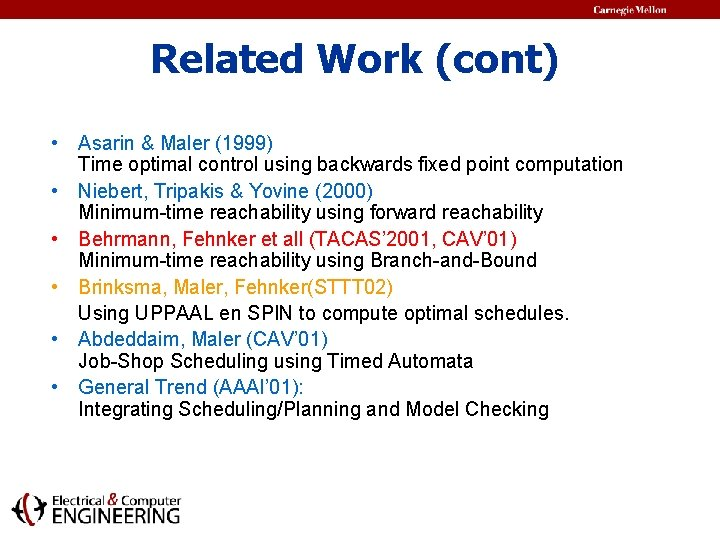Related Work (cont) • Asarin & Maler (1999) Time optimal control using backwards fixed point computation • Niebert, Tripakis & Yovine (2000) Minimum-time reachability using forward reachability • Behrmann, Fehnker et all (TACAS’ 2001, CAV’ 01) Minimum-time reachability using Branch-and-Bound • Brinksma, Maler, Fehnker(STTT 02) Using UPPAAL en SPIN to compute optimal schedules. • Abdeddaim, Maler (CAV’ 01) Job-Shop Scheduling using Timed Automata • General Trend (AAAI’ 01): Integrating Scheduling/Planning and Model CheckingEnd of slide show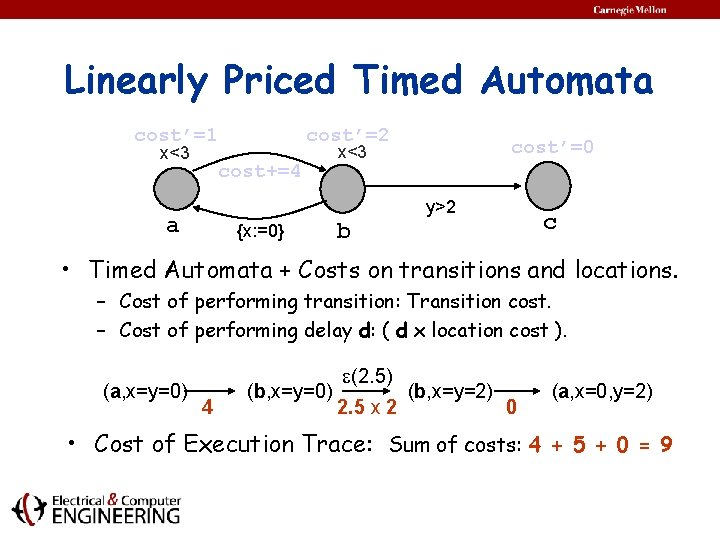Linearly Priced Timed Automata cost’=1 x<3 cost’=2 cost+=4 cost’=0 x<3 y>2 a {x: =0} c b • Timed Automata + Costs on transitions and locations. – Cost of performing transition: Transition cost. – Cost of performing delay d: ( d x location cost ). (a, x=y=0) 4 (b, x=y=0) (2. 5) 2. 5 x 2 (b, x=y=2) 0 (a, x=0, y=2) • Cost of Execution Trace: Sum of costs: 4 + 5 + 0 = 9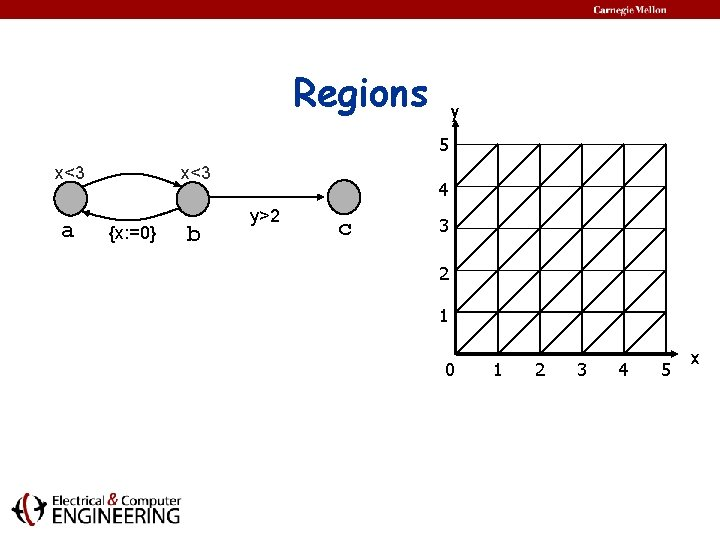Regions y 5 x<3 a x<3 {x: =0} b 4 y>2 c 3 2 1 0 1 2 3 4 5 xRegions y 5 x<3 a x<3 {x: =0} b 4 y>2 c 3 2 1 0 1 2 3 4 5 x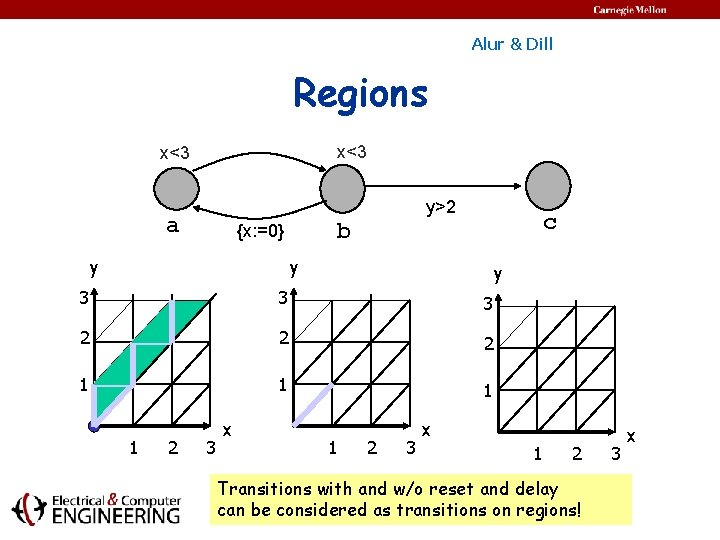Alur & Dill Regions x<3 y>2 a y c b {x: =0} y y 3 3 3 2 2 2 1 1 2 3 x 1 2 Transitions with and w/o reset and delay can be considered as transitions on regions! 3 x